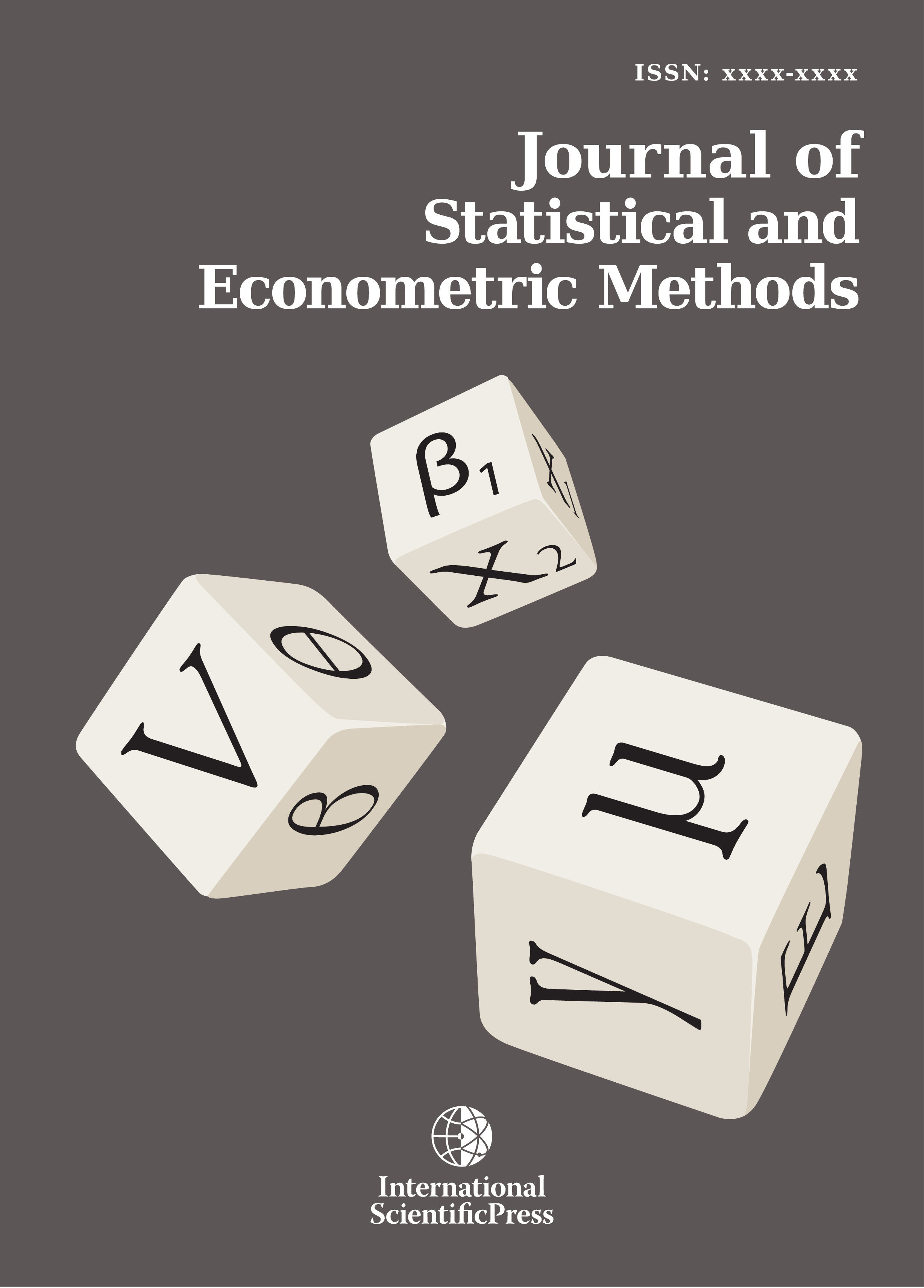# Journal of Statistical and Econometric Methods

#### Modeling for Regressing Variables

•[ Download ]
• Times downloaded: 1818
• Abstract

Regression modeling is an approach to modeling the relationship between endogenous and one or more explanatory exogenous variables. This paper reviews the bias of this modeling, as well as history, purpose, assumptions, and steps. Then the paper follows with Classification of regression analysis in to 8 classes. Specifically, linear and non linear regression is discussed by details. We end the paper with application of regression modeling. We find this paper as a statistical modeling guide for scholars.ISSN: 2241-0376 (Online)
2241-0384 (Print)